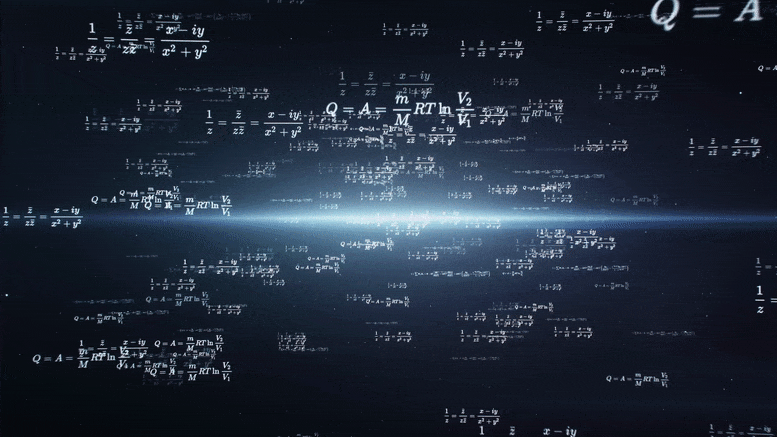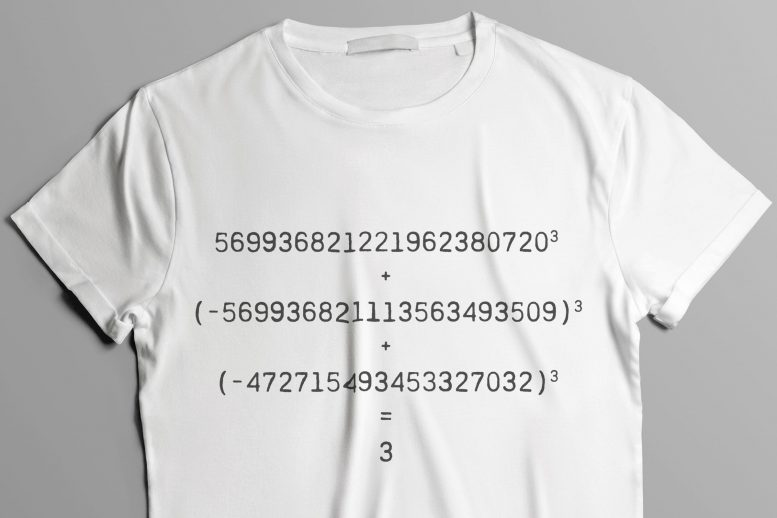# After Cracking the “Sum of Cubes” Puzzle for 42, Mathematicians Solve Harder Problem That Has Stumped Experts for DecadesThe 21-digit solution to the decades-old problem suggests many more solutions exist.

What do you do after solving the answer to life, the universe, and everything? If you’re mathematicians Drew Sutherland and Andy Booker, you go for the harder problem.

In 2019, Booker, at the University of Bristol, and Sutherland, principal research scientist at MIT, were the first to find the answer to 42. The number has pop culture significance as the fictional answer to “the ultimate question of life, the universe, and everything,” as Douglas Adams famously penned in his novel “The Hitchhiker’s Guide to the Galaxy.” The question that begets 42, at least in the novel, is frustratingly, hilariously unknown.

In mathematics, entirely by coincidence, there exists a polynomial equation for which the answer, 42, had similarly eluded mathematicians for decades. The equation x3+y3+z3=k is known as the sum of cubes problem. While seemingly straightforward, the equation becomes exponentially difficult to solve when framed as a “Diophantine equation” — a problem that stipulates that, for any value of k, the values for x, y, and z must each be integers.

When the sum of cubes equation is framed in this way, for certain values of k, the integer solutions for x, y, and z can grow to enormous numbers. The number space that mathematicians must search across for these numbers is larger still, requiring intricate and massive computations.

Over the years, mathematicians had managed through various means to solve the equation, either finding a solution or determining that a solution must not exist, for every value of k between 1 and 100 — except for 42.In September 2019, researchers, harnessing the combined power of half a million home computers around the world, for the first time found a solution to 42. The widely reported breakthrough spurred the team to tackle an even harder, and in some ways more universal problem: finding the next solution for 3. Credit: Christine Daniloff, MIT

In September 2019, Booker and Sutherland, harnessing the combined power of half a million home computers around the world, for the first time found a solution to 42. The widely reported breakthrough spurred the team to tackle an even harder, and in some ways more universal problem: finding the next solution for 3.

Booker and Sutherland have now published the solutions for 42 and 3, along with several other numbers greater than 100, recently in the Proceedings of the National Academy of Sciences.

#### Picking up the gauntlet

The first two solutions for the equation x3+y3+z3 = 3 might be obvious to any high school algebra student, where x, y, and z can be either 1, 1, and 1, or 4, 4, and -5. Finding a third solution, however, has stumped expert number theorists for decades, and in 1953 the puzzle prompted pioneering mathematician Louis Mordell to ask the question: Is it even possible to know whether other solutions for 3 exist?

“This was sort of like Mordell throwing down the gauntlet,” says Sutherland. “The interest in solving this question is not so much for the particular solution, but to better understand how hard these equations are to solve. It’s a benchmark against which we can measure ourselves.”

As decades went by with no new solutions for 3, many began to believe there were none to be found. But soon after finding the answer to 42, Booker and Sutherland’s method, in a surprisingly short time, turned up the next solution for 3:

5699368212219623807203 + (−569936821113563493509)3 + (−472715493453327032)3 = 3

The discovery was a direct answer to Mordell’s question: Yes, it is possible to find the next solution to 3, and what’s more, here is that solution. And perhaps more universally, the solution, involving gigantic, 21-digit numbers that were not possible to sift out until now, suggests that there are more solutions out there, for 3, and other values of k.

“There had been some serious doubt in the mathematical and computational communities, because [Mordell’s question] is very hard to test,” Sutherland says. “The numbers get so big so fast. You’re never going to find more than the first few solutions. But what I can say is, having found this one solution, I’m convinced there are infinitely many more out there.”

#### A solution’s twist

To find the solutions for both 42 and 3, the team started with an existing algorithm, or a twisting of the sum of cubes equation into a form they believed would be more manageable to solve:

k − z3 = x3 + y3 = (x + y)(x2 − xy + y2)

This approach was first proposed by mathematician Roger Heath-Brown, who conjectured that there should be infinitely many solutions for every suitable k. The team further modified the algorithm by representing x+y as a single parameter, d. They then reduced the equation by dividing both sides by d and keeping only the remainder — an operation in mathematics termed “modulo d” — leaving a simplified representation of the problem.

“You can now think of k as a cube root of z, modulo d,” Sutherland explains. “So imagine working in a system of arithmetic where you only care about the remainder modulo d, and we’re trying to compute a cube root of k.”

With this sleeker version of the equation, the researchers would only need to look for values of d and z that would guarantee finding the ultimate solutions to x, y, and z, for k=3. But still, the space of numbers that they would have to search through would be infinitely large.

So, the researchers optimized the algorithm by using mathematical “sieving” techniques to dramatically cut down the space of possible solutions for d.

“This involves some fairly advanced number theory, using the structure of what we know about number fields to avoid looking in places we don’t need to look,” Sutherland says.

The team also developed ways to efficiently split the algorithm’s search into hundreds of thousands of parallel processing streams. If the algorithm were run on just one computer, it would have taken hundreds of years to find a solution to k=3. By dividing the job into millions of smaller tasks, each independently run on a separate computer, the team could further speed up their search.

In September 2019, the researchers put their plan in play through Charity Engine, a project that can be downloaded as a free app by any personal computer, and which is designed to harness any spare home computing power to collectively solve hard mathematical problems. At the time, Charity Engine’s grid comprised over 400,000 computers around the world, and Booker and Sutherland were able to run their algorithm on the network as a test of Charity Engine’s new software platform.

“For each computer in the network, they are told, ‘your job is to look for d’s whose prime factor falls within this range, subject to some other conditions,’” Sutherland says. “And we had to figure out how to divide the job up into roughly 4 million tasks that would each take about  three hours for a computer to complete.”

Very quickly, the global grid returned the very first solution to k=42, and just two weeks later, the researchers confirmed they had found the third solution for k=3 — a milestone that they marked, in part, by printing the equation on t-shirts.

The fact that a third solution to k=3 exists suggests that Heath-Brown’s original conjecture was right and that there are infinitely more solutions beyond this newest one. Heath-Brown also predicts the space between solutions will grow exponentially, along with their searches. For instance, rather than the third solution’s 21-digit values, the fourth solution for x, y, and z will likely involve numbers with a mind-boggling 28 digits.

“The amount of work you have to do for each new solution grows by a factor of more than 10 million, so the next solution for 3 will need 10 million times 400,000 computers to find, and there’s no guarantee that’s even enough,” Sutherland says. “I don’t know if we’ll ever know the fourth solution. But I do believe it’s out there.”

Reference: “On a question of Mordell” by Andrew R. Booker and Andrew V. Sutherland, 10 March 2021, Proceedings of the National Academy of Sciences.
DOI: 10.1073/pnas.2022377118

This research was supported, in part, by the Simons Foundation.

[Editor’s Note (March 23, 2021): A reference to “whole numbers” in the article was replaced with “integers” as negative values are allowed.]

#### 8 Commentson "After Cracking the “Sum of Cubes” Puzzle for 42, Mathematicians Solve Harder Problem That Has Stumped Experts for Decades"

1.Muhammad | March 21, 2021 at 3:17 pm | Reply

ALLAH

2.Alexiev | March 21, 2021 at 6:16 pm | Reply

42 is the answer. Who didn’t know that? A “make work” project of little consequence to anyone, with the possible exception of math geeks. What I’m more concerned about is how one gains access to the processing capacity of a half-million computers, worldwide… RIGHT! Through the “Cloud”. I wonder: Since ITT, ATT and IBM now control that resource, do they still have permissions in place, or are said permissions a “programmed-in” feature in all computers and cellphones? Hmm… Are 5G and Micro-trading coincident? 🤔 Something to think about while awaiting a cure for THE COVID…

3.Jason Cortese | March 23, 2021 at 2:22 am | Reply

How can x,y and z all be whole numbers as separate variables if x,y and z are all one whole set since they all exist together as one absolute set.

4.Jeremy | March 23, 2021 at 8:41 am | Reply

I almost stopped reading when paragraph 3 said “the values for x, y, and z must each be whole numbers”, and the very next paragraph said they are integer solutions.

•Mike O'Neill | March 23, 2021 at 9:42 am | Reply

Thanks for your comment. “Whole numbers” in that paragraph has been replaced with “integers” as negative numbers are allowed.

5.Dawn | March 24, 2021 at 3:33 am | Reply

Wont ever be satisfied effectively until 4th variant is factored in. Though, yes, you can get a result for this equation. (Obviously) However….triangles lead to squares and so on down the line.

6.Prem Pratap Singh | November 26, 2021 at 1:39 am | Reply

The paper ” A Discovery of the taxi cab number 1729″ published by research india publication proves that we can separate a cube as a sum of three different cubes .

•Roh | December 13, 2021 at 6:12 pm | Reply

Not really relevant here since the examples of k being used are not cubes.